Courses

# Linear Algebra (Part - 1) Civil Engineering (CE) Notes | EduRev

## GATE : Linear Algebra (Part - 1) Civil Engineering (CE) Notes | EduRev

The document Linear Algebra (Part - 1) Civil Engineering (CE) Notes | EduRev is a part of the GATE Course Topic wise GATE Past Year Papers for Civil Engineering.
All you need of GATE at this link: GATE

Q.1. Consider the hemispherical tank of radius 13 m as shown in the figure (not drawn to scale). What is the volume of water (in m3) when the depth of water at the centre of the tank is 6 m?
[2019: 2 Marks, Set-ll]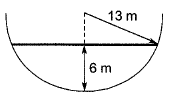(a) 156π
(b) 396π
(c) 468π
(d) 78π

Ans. (b)
Solution. Volume of water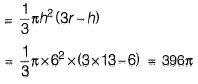Q.2. The inverse of the matrix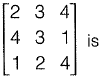[2019: 2 Marks, Set-ll]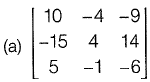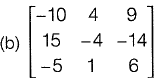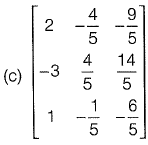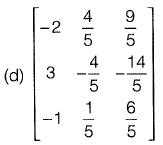Ans. (d)
Q.3. The rank of the following matrix is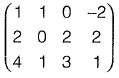[2018 : 2 Marks, Set-II]
(a) 1
(b) 2
(c) 3
(d) 4
Ans. (b)
Solution.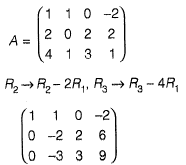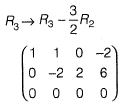► Number of non zero rows = 2
► Rank of A = 2

Q.4. The matrix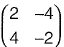has
[2018 : 2 Marks, Set-II]
(a) real eigenvalues and eigenvectors
(b) real eigenvalues but complex eigenvectors
(c) complex eigenvalues but real eigenvectors
(d) complex eigenvalues and eigenvectors
Ans. (d)
Solution.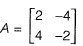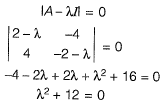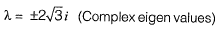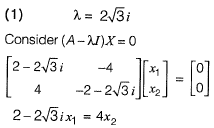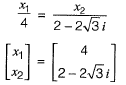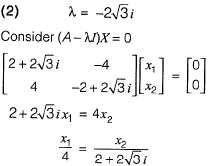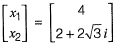Complex Eigenvalues and complex Eigen vectors.

Q.5. Which one of the following matrices is singular?
[2018: 1 Marks, Set-I]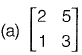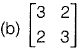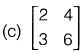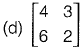Ans. (c)
Solution. Option (a): |A| = 6 - 5 = 1
Option (b): |A| = 9 - 4 = 5
Option (c): |A| = 12-12 = 0
Option (d): |A| = 8 - 18 = -10
Hence matrix (c) is singular.

Try yourself:For the given orthogonal matrix Q,    [2018: 1 Marks, Set-I]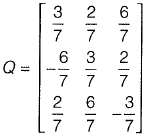The inverse is

Q.7. If  A =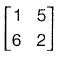and B =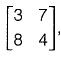ABT is equal to
[2017 : 2 Marks, Set-II]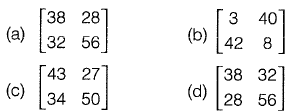Ans. (a)
Solution.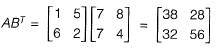Q.8. Consider the following simultaneous equations (with c1 and c2 being constants):
[2017 : 1 Mark, Set-II]
3x1 + 2x2 = c1
4x1 + x2 = c2
The characteristics equation for these simultaneous equations is
(a) λ2 - 4λ - 5 = 0
(b) λ2 - 4λ + 5 = 0
(c) λ2 + 4λ - 5 = 0
(d) λ2 + 4λ + 5 = 0
Ans. (a)
Solution.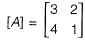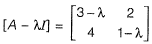|A - λI| = 0
(3 - λ) (1 - λ) - 8 = 0
3 - 4λ + λ2 - 8 = 0
λ2 - 4λ - 5 = 0

Try yourself:Consider the matrix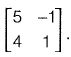Which one of the following statements is TRUE for the eigenvalues and eigenvectors of this matrix?    [2017: 2 Marks, Set-I]

Q.10. The matrix P is the inverse of a matrix Q. If I denotes the identity matrix, which one of the following options is correct?  [2017: 1 Mark, Set-I]
(a) PQ = I but QP ≠ I
(b) QP = I but PQ ≠ I
(c) PQ = I and QP = I
(d) PQ = QP = I
Ans. (c)
Solution. Given that P is inverse of Q.
► P = Q-1
► PQ = Q-1Q,  QP = QQ-1
► PQ = I , QP = I
∴ PQ = QP = I

Try yourself:Consider the following linear system.    [2016 : 2 Marks, Set-Il]
x + 2y - 3z = a
2x + 3y + 3x = b
5x + 9y - 6z = c
This system is consistent if a, b and c satisfy the equation

Try yourself:If the entries in each column of a square matrix M add up to 1, then an eigen value of M is [2016 : 1 Mark, Set - I]

Try yourself:The two Eigenvalues of the matrix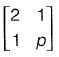have a ratio of 3 : 1 for p = 2. What is another value of p for which the Eigenvalues have the same ratio of 3 : 1?
[2015: 2 Marks, Set-II]

Try yourself:Let A =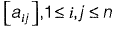with n > 3 and aij = i.j. The rank of A is    [2015 : 1 Mark, Set-II]

Try yourself:The smallest and largest Eigen values of the following matrix are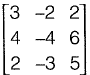[2015 : 2 Marks, Set-I]

Question 16: For what value of p the following set of equations will have no solution?    [2015 : 1 Mark, Set-I]
2x + 3y = 5
3x + py = 10
Solution:
Given system of equations has no solution if the lines are parallel i.e., their slopes are equal
2/3 = 3/p
⇒ p = 4.5
Question 17: The rank of the matrix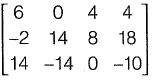is _____.    [2014 : 2 Marks, Set-II]
Solution: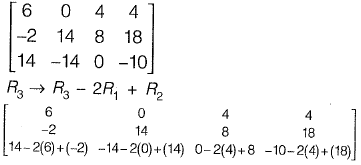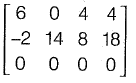Determinant of matrix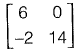is not zero.
∴ Rank is 2
Question 18: The determinant of matrix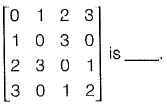[2014 : 1 Mark, Set-II]
Solution: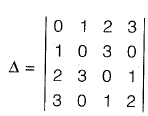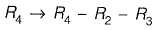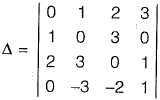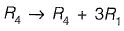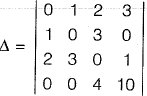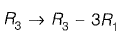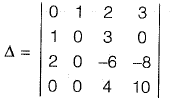Interchanging column 1 and column 2 and taking transpose,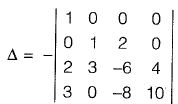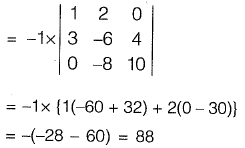Question 19: With reference to the conventional Cartesian (x, y) coordinate system, the vertices of a triangle have the following coordinates; (x1, y1) = (1, 0); (x2, y2) = (2, 2); (x3, y3) = (4, 3). The area of the triangle is equal to    [2014 : 1 Mark, Set-I]
(a) 3/2
(b) 3/4
(c) 4/5
(d) 5/2
Solution: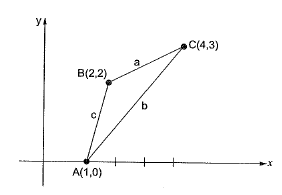Area of triangle is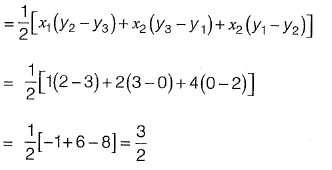Question 20: The sum of Eigen values of matrix, [M] is where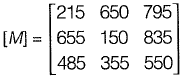[2014 : 1 Mark, Set-I]
(a) 915
(b) 1355
(c) 1640
(d) 2180
Solution: Sum of eigen values = trace of matrix
= 215 + 150 + 550 = 915

Question 21: Given the matrices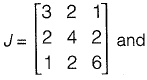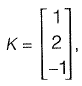the product KT JK is ____.    [2014 : 1 Mark, Set-I]
Solution: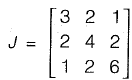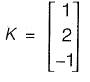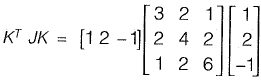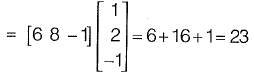Question 22: There are three matrixes P(4 x 2), Q(2 x 4) and R(4 x 1). The minimum of multiplication required to compute the matrix PQR is    [2013 : 1 Mark]
Solution: If we multiply QR first then,
Q2x4 x R(4x1) having multiplication number 8.
There fore P(4 x 2) QR(2 x 1) will have minimum number of multiplication = (8 + 8) = 16.

Question 23: The eigen values of matrix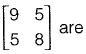[2011 : 2 Marks]
(a) -2.42 and 6.86
(b) 3.48 and 13.53
(c) 4.70 and 6.86
(d) 6.86 and 9.50
Solution: We need eigen values of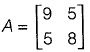The characteristic equation is,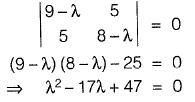So eigen values are,
λ = 3.48, 13.53

Question 24: [A] is square matix which is neither symmetric nor skew-symmetric and [A]T is its transpose. The sum and difference of these matrices are defined as [S] = [A] + [A]T and [D] = [A] - [A]T, respectively. Which of the following statements is TRUE?    [2011 : 1 Mark]
(a) Both [S] and [D] are symmetric
(b) Both [S] and [D] are skew-symmetric
(c) [S] is skew-symmetric and [D] is symmetric
(d) [S] is symmetric and [D] is skew-symmetric
Solution: Since (A + At) = At + (At)t
= At + A
i.e. St = S
∴ S is symmetric
Since (A - At)t = At - (At)t
= At - A = -(A - At)
i.e. Dt = - D
So D is Skew-Symmetric.

Question 25: The inverse of the matrix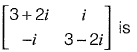[2010 : 2 Marks]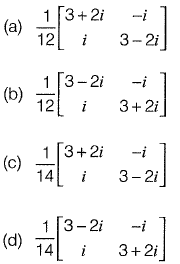Solution: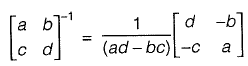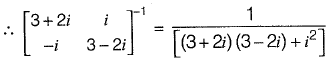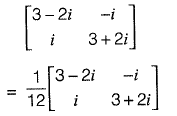Offer running on EduRev: Apply code STAYHOME200 to get INR 200 off on our premium plan EduRev Infinity!

67 docs

,

,

,

,

,

,

,

,

,

,

,

,

,

,

,

,

,

,

,

,

,

;